# Python 数据分析学习笔记（一）：Pandas 入门

Python 数据分析学习笔记（一）：Pandas 入门

# 一、Pandas 概述

1. 概述

Pandas 是数据分析的三大剑客之一，是 Python 的核心数据分析库，它提供了快速、灵活、明确的数据结构，能够简单、直观、快速地处理各种类型的数据，具体介绍如下：Pandas 能够处理以下类型的数据：

1. 与 SQL 或 Excel 表类似的数据。
2. 有序和无序 (非固定频率) 的时间序列数据。
3. 带行列标签的矩阵数据。
4. 任意其他形式的观测、统计数据集。

Pandas 提供的两个主要数据结构 Series(一维数组结构) 与 DataFrame(二维数组结构)，可以处理金融、统计、社会科学、工程等领域里的大多数典型案例，并且 Pandas 是基于 NumPy 开发的，它可以与其他第三方科学计算库完美集成。Pandas 的功能很多，它的优势如下：

1. 处理浮点与非浮点数据里的缺失数据，表示为NaN。
2. 大小可变，例如插入或删除 DataFrame 等多维对象的列。
3. 自动、显式数据对齐，显式地将对象与一组标签对齐，也可以忽略标签，在 Series、DataFrame 计算时自动与数据对齐。
4. 强大、灵活的分组统计(groupby) 功能，即数据聚合、数据转换。
5. 可以把 Python 和 NumPy 数据结构里不规则、不同索引的数据轻松地转换为 DataFrame 对象。
6. 智能标签，对大型数据集进行切片、花式索引、子集分解等操作。
7. 直观地合并 (merge)、连接(join) 数据集。
8. 灵活地重塑 (reshape)、透视 (pivot) 数据集。
9. 成熟的导入导出工具，导入文本文件(CSV 等支持分隔符的文件)、Excel 文件、数据库等来源的数据；导出 Excel 文件、文本文件等，利用超快的 HDF5 格式保存或加载数据。
10. 时间序列：支持日期范围生成、频率转换、移动窗口统计、移动窗口线性回归、日期位移等时间序列功能。

2. 安装 Pandas

``````pip install xlrd
pip install xlwt
pip install Pandas
``````

# 二、Series 对象

Series 是 Python 的 Pandas 库中的一种数据结构，它类似一维数组，由一组数据以及这组数据相关的标签 (即索引) 组成，或者仅有一组数据而没有索引也可以创建一个简单的 Series 对象。Series 可以存储整数、浮点数、字符串、Python 对象等多种类型的数据。1. 创建一个 Series 对象

``````s=pd.Series(data,index=index)
``````

1. data：表示数据，支持 Python 字典、多维数组、标量值 (即只有大小、没有方向的量。也就是说，只是一个数值，如 s=pd.Series(5))。
2. index：表示行标签(索引)。
3. 返回值：Series 对象。

2. 创建一个 Series 对象，为成绩表添加一列物理成绩，程序代码如下：3. 手动设置索引4. Series 的索引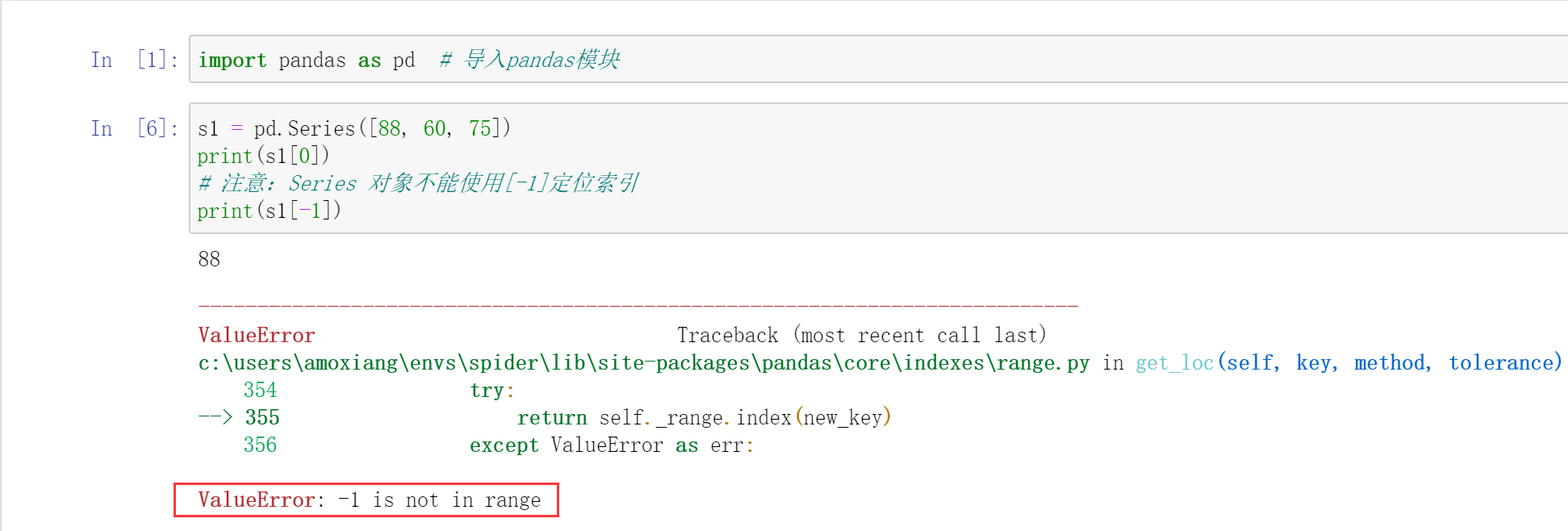Series 标签索引与位置索引方法类似，用 `[]` 表示，里面是索引名称，注意 index 的数据类型是字符串，如果需要获取多个标签索引值，则用 `[[]]` 表示（相当于在 `[]` 中包含一个列表）。通过标签索引 `向同学``刘同学` 获取物理成绩，程序代码如下：5. Series 的切片索引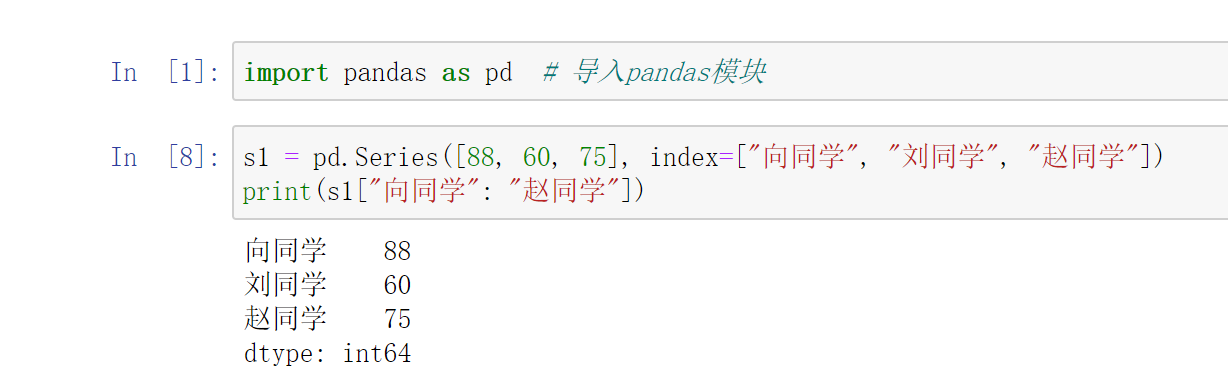6. 获取 Series 的索引和值# 三、DataFrame 对象

DataFrame 是 Pandas 库中的一种数据结构，它是由多种类型的列组成的二维表数据结构，类似于 Excel、SQL 或 Series 对象构成的字典。DataFrame 是最常用的 Pandas 对象，它与 Series 对象一样支持多种类型的数据。

1. 图解 DataFrame 对象

DataFrame 是一个二维表数据结构，即由行列数据组成的表格。DataFrame 既有行索引也有列索引，它可以看作是由 Series 对象组成的字典，不过这些 Series 对象共用一个索引，如下图所示。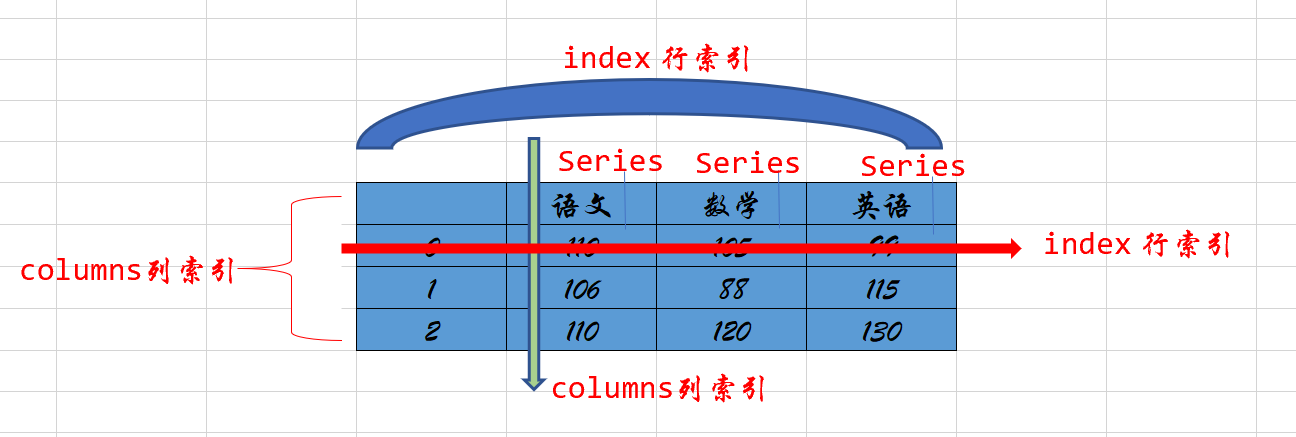2. 创建一个 DataFrame 对象

``````pandas.DataFrame(data, index, columns, dtype, copy)
``````

1. data：表示数据，可以是 ndarray 数组、series 对象、列表、字典等。
2. index：表示行标签(索引)。
3. columns：列标签(索引)。
4. dtype：每一列数据的数据类型，其与 Python 数据类型有所不同，如 object 数据类型对应的是 Python 的字符型。如下表所示，是 Pandas 数据类型与 Python 数据类型的对应。5. copy：用于复制数据。
6. 返回值：DataFrame。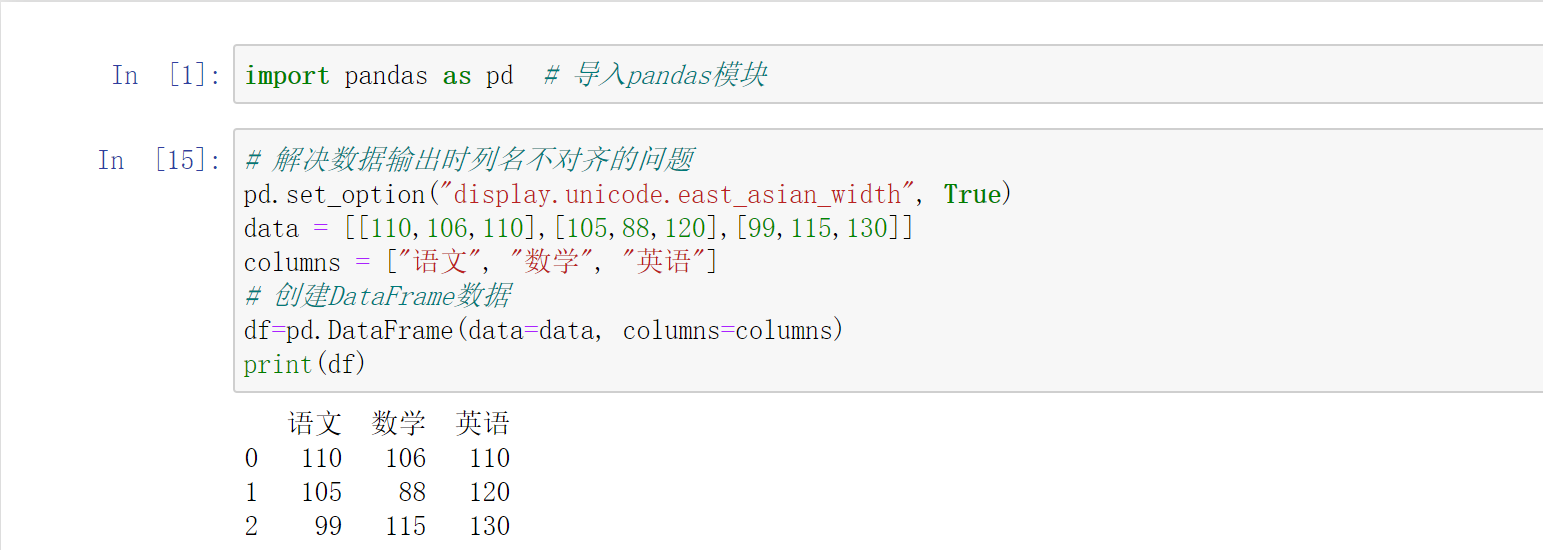3. DataFrame 的重要属性和函数

DataFrame 是 Pandas 中一个重要的对象，它的属性和函数有很多，下面先简单了解一下 DataFrame 对象的几个重要属性和函数，重要属性及描述如下表所示：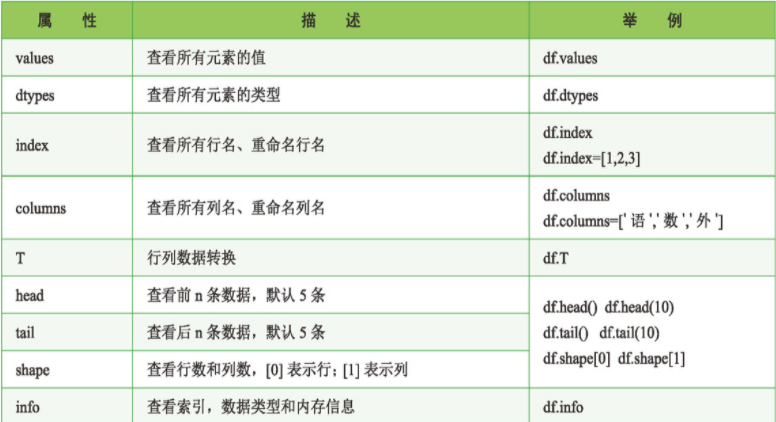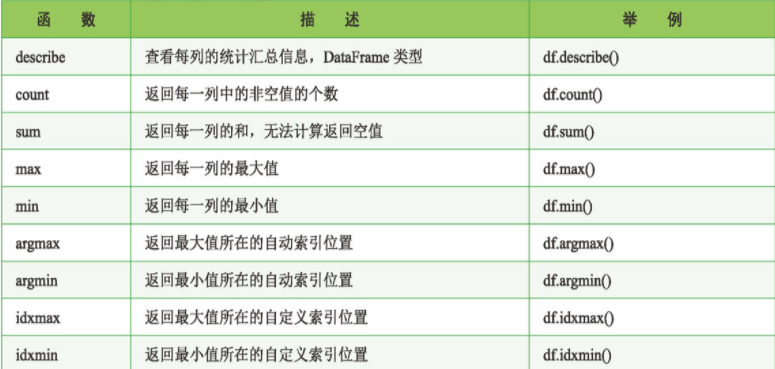# 四、导入外部数据

## 1. 导入 .xls 或 .xlsx 文件

``````def read_excel(
index_col=None, usecols=None, squeeze=False, dtype=None,
engine=None, converters=None, true_values=None, false_values=None,
skiprows=None, nrows=None, na_values=None, keep_default_na=True,
na_filter=True, verbose=False, parse_dates=False, date_parser=None,
thousands=None, comment=None, skipfooter=0, convert_float=True,
mangle_dupe_cols=True,
):
``````

1. io：字符串，xls或 xlsx 文件路径或类文件对象。
2. sheet_name：None、字符串、整数、字符串列表或整数列表，默认值为 0。字符串用于工作表名称；整数为索引，表示工作表位置，字符串列表或整数列表用于请求多个工作表，为 None 时则获取所有的工作表。参数值如下表所示。4. names：默认值为None，要使用的列名列表。
5. index_col：指定列为索引列，默认值为 None，索引 0 是 DataFrame 对象的行标签。
6. usecols：int、list 或字符串，默认值为 None。
• 如果为 None，则解析所有列。
• 如果为 int，则解析最后一列。
• 如果为 list 列表，则解析列号和列表的列。
• 如果为字符串，则表示以逗号分隔的 Excel 列字母和列范围列表(例如，A : E 或 A, C, E : F)，范围包括双方。
7. squeeze：布尔值，默认值为 False，如果解析的数据只包含一列，则返回一个 Series。
8. dtype：列的数据类型名称或字典，默认值为 None。例如， 为 { ‘a’ : np.float64，‘b’ : np.int32}。
9. skiprows：省略指定行数的数据，从第一行开始。
10. skipfooter：省略指定行数的数据，从尾部数的行开始。

1. 常规导入• `../`：表示当前文件所在目录的上一级目录。
• `./`：表示当前文件所在的目录 (可以省略)。
• `/`：表示当前文件的根目录 (域名映射或硬盘目录)。

2. 导入指定的 Sheet 页3. 通过行列索引导入指定行列数据

DataFrame 是二维数据结构，因此它既有行索引又有列索引。当导入 Excel 数据时，行索引会自动生成，如 0、1、2，而列索引则默认将第 0 行作为列索引(如 A，B，…，J)。如果通过指定行索引导入 Excel 数据，则需要设置 index_col 参数。下面将 `买家会员名` 作为行索引(位于第 0 列)，导入 Excel 数据，程序代码如下：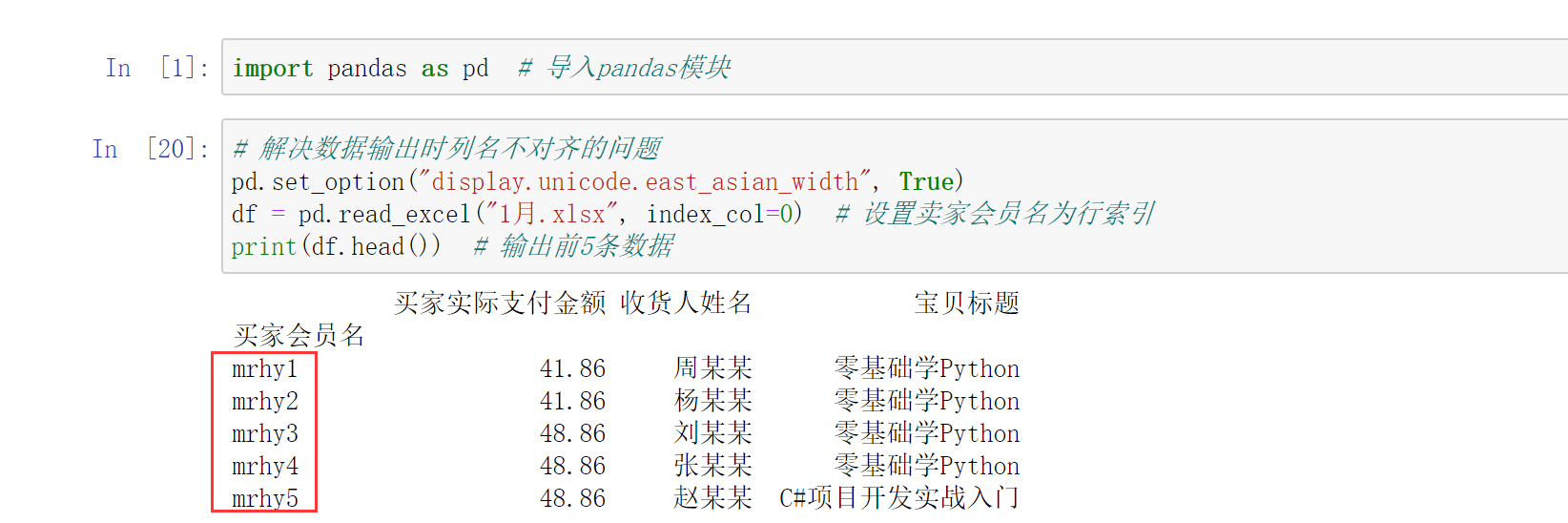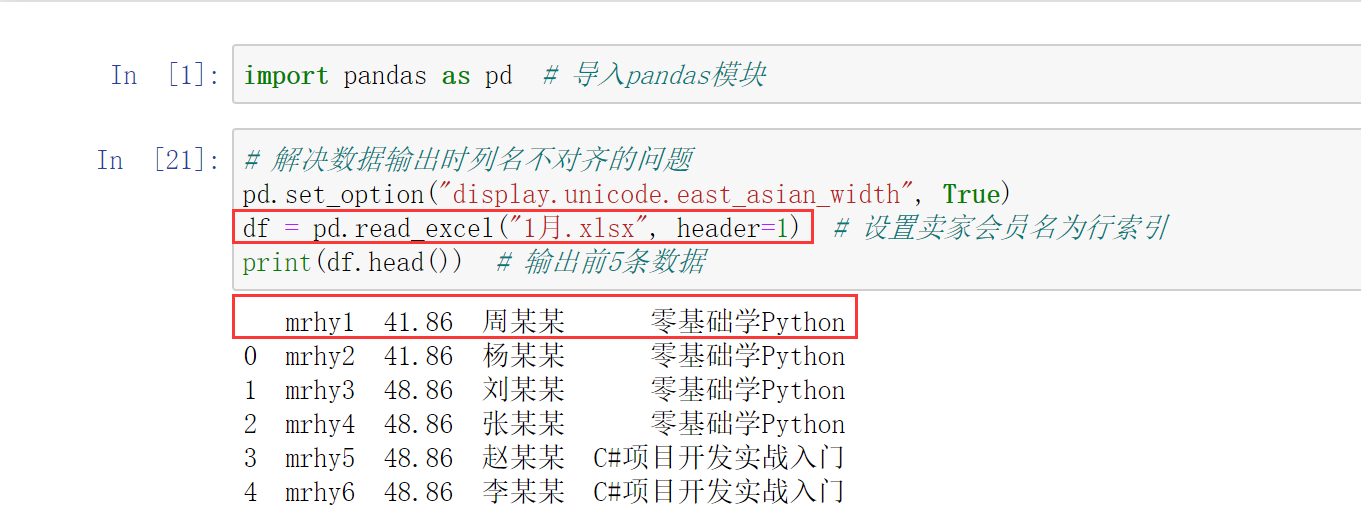4. 导入指定列数据``````df = pd.read_excel("1月.xlsx", usecols=[0,3])
``````

``````df = pd.read_excel("1月.xlsx", usecols=["买家会员名", "宝贝标题"])
``````

## 2. 导入 .csv 文件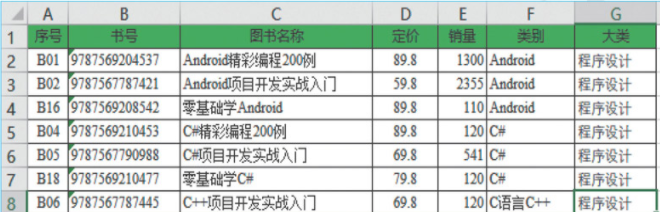csv 文件可以使用记事本打开，可以使用 Excel 另存为 .csv 文件格式，或者在文本编辑器中输入文本，保存为 .csv 文件格式。csv 文件是比较简单的文件格式，缺少 Excel 电子表格的许多功能。例如：

1. 值没有类型，所有数据都是字符串。
2. 没有字体大小和颜色的设置。
3. 没有多个工作表。
4. 不能指定单元格的宽度和高度。
5. 不能合并单元格。
6. 不能嵌入图像或图表。

csv 文件被应用程序广泛支持的原因，也正因为它的简单。它可以在文本编辑器中查看（包括 IDLE 的文件编辑器)，是表示电子表格数据的直接方式。导入 .csv 文件时主要使用 Pandas 的 read_csv() 方法，语法如下：

``````pandas.read_csv(参数省略....) 具体的参数可以点源码进去查看
``````

1. filepath_or_buffer：字符串，文件路径，也可以是 URL 链接。
2. sep、 delimiter：字符串，分隔符。
4. names：默认值为 None, 要使用的列名列表。
5. index_col：指定列为索引列，默认值为 None, 索引 0 是 DataFrame 对象的行标签。
6. usecols：int、 list 或字符串，默认值为 None。
• 如果为 None，则解析所有列。
• 如果为 int，则解析最后一列。
• 如果为 list 列表，则解析列号、列表的列。.
• 如果为字符串，则表示以逗号分隔的 Excel 列字母和列范围列表(例如，A : E 或 A, C, E : F")，范围包括双方。
7. dtype：列的数据类型名称或字典，默认值为 None。例如，{‘a’: np.float64, ‘b’: np.int32}。
8. parse_ dates：布尔类型值、int 类型值的列表、列表或字典，默认值为 False。可以通过 parse_ dates 参数直接将某列转换成 datetime64 的日期类型。例如，df1=pd.read_csv(‘1 月.csv’, parse_dates=[‘订单付款时间"D’])。
• parse_dates为 True 时，尝试解析索引。
• parse_dates 为 int 类型值组成的列表时，如[1,2,3]，则解析 1、2、3 列的值作为独立的日期列。
• parse_date 为列表组成的列表，如[[1,3]]，则将 1、3 列合并，作为一个日期列使用。
• parse_date 为字典时，如{‘总计’: [1,3]}， 则将 1、3 列合并，合并后的列名为总计。
9. encoding：字符串，默认值为 None，文件的编码格式。
10. 返回值：返回一个 DataFrame 对象。

1. 导入.csv 文件## 3. 导入 .txt 文本文件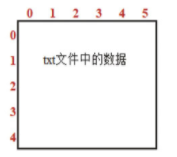## 4. 导入 HTML 网页

1. io：字符串，文件路径，也可以是 URL 链接。网址不接受 https, 可以尝试去掉 https 中的 s 后爬取。
2. match：正则表达式，返回与正则表达式匹配的表格。
3. flavor：解析器默认为 lxml。
5. index_col：指定行标题对应的列，列表 list 为多重索引。
6. encoding：字符串，默认为 None，文件的编码格式。
7. 返回值：返回一个 DataFrame 对象。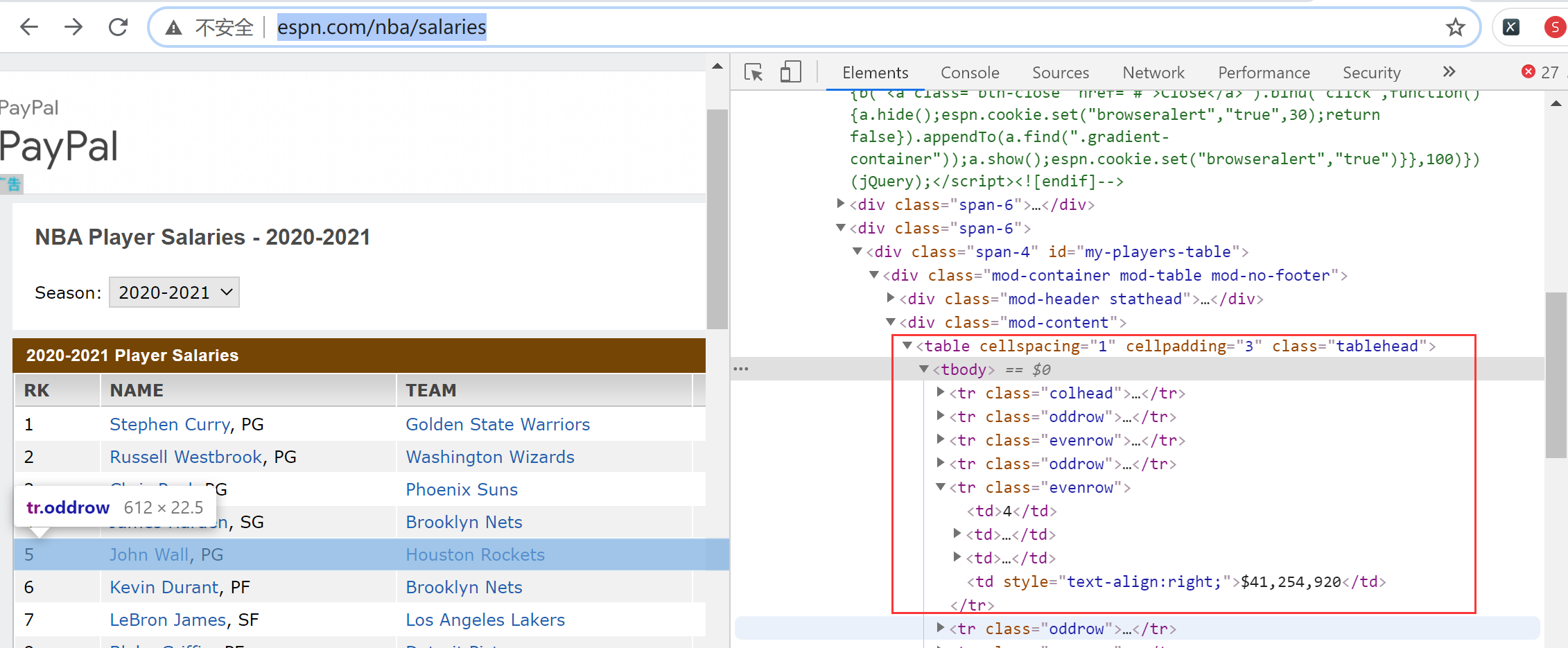# 五、数据抽取DataFrame 对象中的 loc 属性和 iloc 属性都可以抽取数据，区别如下：

1. loc 属性：以列名 (columns) 和行名 (index) 作为参数，当只有一个参数时，默认是行名，即抽取整行数据，包括所有列，如 df.loc[‘A’]
2. iloc 属性：以行和列位置索引(即0,1,2, …作为参数，0 表示第一行；1 表示第二行，以此类推。当只有一个参数时，默认是行索引，即抽取整行数据，包括所有列，如抽取第一行数据，df.iloc。

1. 抽取一行数据2. 抽取多行数据

``````print(df.loc[["明日", "高袁圆"]])
print(df.iloc[[0,2]])
``````

``````print(df.loc["明日":"二月二"])  # 明日到二月二
print(df.loc[:"七月流火":])  # 第1行到七月流火
print(df.iloc[0:4]) # 第1行到第4行
print(df.iloc[1::]) # 第2行到最后一行
``````

3. 抽取指定列数据``````print(df.loc[:,["语文","数学"]])  # 抽取语文和数学
print(df.iloc[:,[0,1]])  # 抽取第1列和第2列
print(df.loc[:,"语文":])  # 抽取从"语文"开始到最后一列
print(df.iloc[:,:2])  # 连续抽取从第1列开始到第3列，但不包括第3列
``````

4. 抽取指定行列数据5. 按指定条件抽取数据

(1) 取其中的一个元素，如 .iat[x,x]。
(2) 基于位置的查询，如 .iloc[]、iloc[2,1]。
(3) 基于行列名称的查询，如 .loc[x]。# 六、数据的增加、修改和删除

## 1. 增加数据1. 按列增加数据

(1) 直接为 DataFrame 对象赋值。(2) 使用 loc 属性在 DataFrame 对象的最后增加一列。

``````df.loc[:, "物理"] = [88,79,60,50]
``````

(3) 在指定位置插入一列。

``````wl = [88,79,60,50]
df.insert(1,"物理",wl)
``````2. 按行增加数据

(1) 增加一行数据。增加一行数据主要使用 loc 属性实现。在成绩表中增加一行数据，即钱多多同学的成绩，关键代码如下：

``````df.loc["钱多多"] = [100,120,99]
``````

(2) 增加多行数据。增加多行数据主要使用字典并结合 append 方法实现。在原有数据中增加钱多多，童年，无名，同学的考试成绩，关键代码如下：

``````df_insert = pd.DataFrame({
"语文":[100,123,138],"数学":[99,142,60],"英语":[98,139,99]}
,index = ["钱多多","童年","无名"])
df1 = df.append(df_insert)
print(df1)
``````

## 2. 修改数据1. 修改列标题

``````df.columns = ["语文", "数学(上)", "英语"]
print(df)
``````

``````df.rename(columns={
"语文":"语文(上)", "数学":"数学(上)", "英语":"英语(上)"},inplace=True)
``````

2. 修改行标题

``````df.index=list("1234")
``````

``````df.rename({
"明日":1,"七月流火":2,"高袁圆":3,"二月二":4},axis=0,inplace=True)
``````

3. 修改数据

(1) 修改整行数据。例如，修改明日同学的各科成绩，关键代码如下：

``````df.loc["明日"] = [120,115,109]
``````

``````df.loc["明日"] = df.loc["明日"] + 10
``````

(2) 修改整列数据。例如，修改所有同学的语文成绩，关键代码如下：

``````df.loc[:,"语文"]=[115,108,112,118]
``````

(3) 修改某一处数据。例如，修改明日同学的语文成绩，关键代码如下：

``````df.loc["明日", "语文"] = 115
``````

(4) 使用 iloc 属性修改数据。通过 iloc 属性指定行列位置实现修改数据，关键代码如下：

``````df.iloc[0,0]=115  # 修改某一处数据
df.iloc[:,0]=[115,108,112,118]  # 修改整列数据
df.iloc[0,:]=[120,115,109]  # 修改整行数据
``````

## 3. 删除数据

``````def drop(self, labels=None, axis=0, index=None,
columns=None, level=None, inplace=False, errors="raise",)
``````

1. labels：表示行标签或列标签。
2. axis：axis = 0，表示按行删除；axis = 1，表示按列删除，默认值为 0。
3. index：删除行，默认值为 None。
4. columns：删除列，默认值为 None。
5. level：针对有两级索引的数据。level = 0，表示按第 1 级索引删除整行；level = 1，表示按第 2 级索引删除整行，默认值为 None。
6. inplace：可选参数，对原数组作出修改并返回一个新数组。默认值为 False，如果值为 True，那么原数组直接就将被替换。
7. errors：参数值为 ignore 或 raise，默认值为 raise，如果值为 ignore(忽略)，则取消错误。

1. 删除行列数据

``````df.drop(["数学"],axis=1,inplace=True)  # 删除某列
df.drop(columns="数学",inplace=True)  # 删除columns为数学的列
df.drop(labels="数学",axis=1,inplace=True)  # 删除列标签为数学的列
df.drop(["明日", "二月二"],inplace=True)  # 删除某一行
df.drop(index="明日",inplace=True)  # 删除index为明日的行
df.drop(labels="明日",axis=0,inplace=True)  # 删除行标签为明日的行
``````

2. 删除特定条件的行

``````df.drop(index=df[df["数学"].isin()].index, inplace=True)  # 删除数学包含分数88的行
df.drop(index=df[df["语文"]<110].index,inplace=True)  # 删除语文小于分数110的行
``````

# 七、数据清洗

## 1. 查看与处理缺失值

1. 查看缺失值

``````import pandas as pd  # 导入pandas模块
print(df)
print(df.info())
``````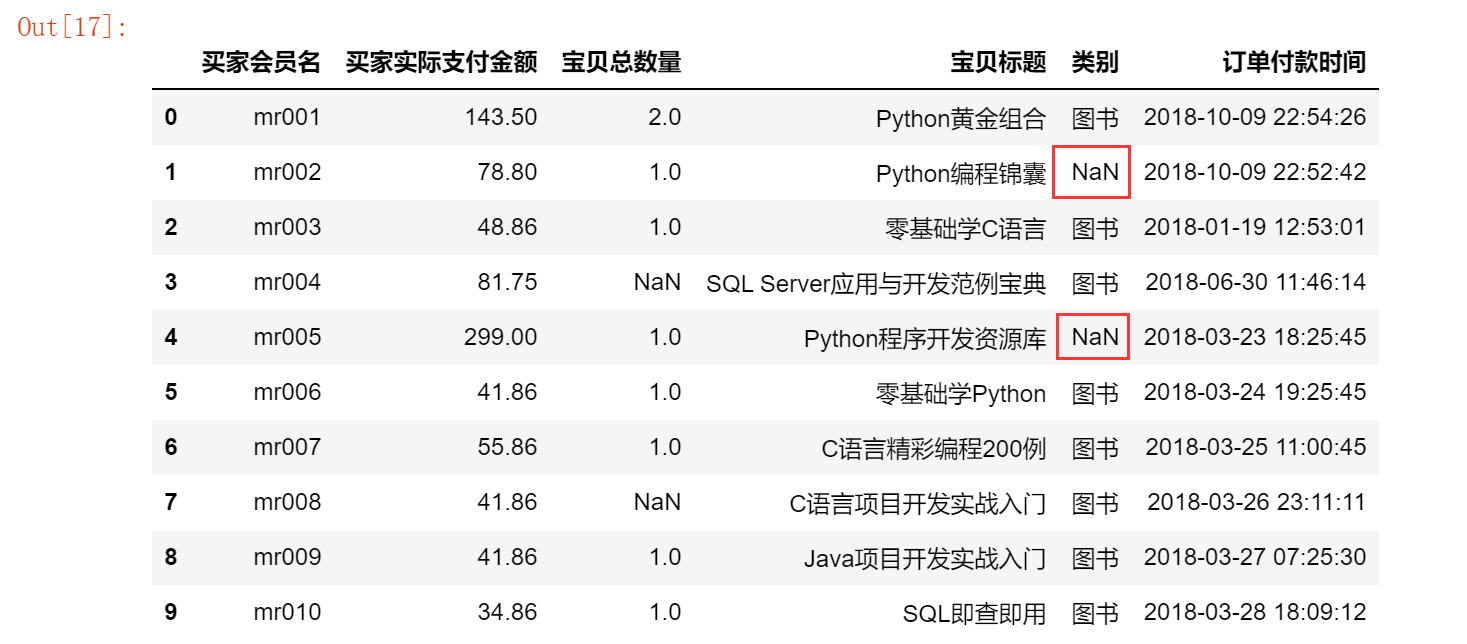``````print(df.isnull())
print(df.notnull())
``````2. 缺失值删除处理

``````df.dropna()
``````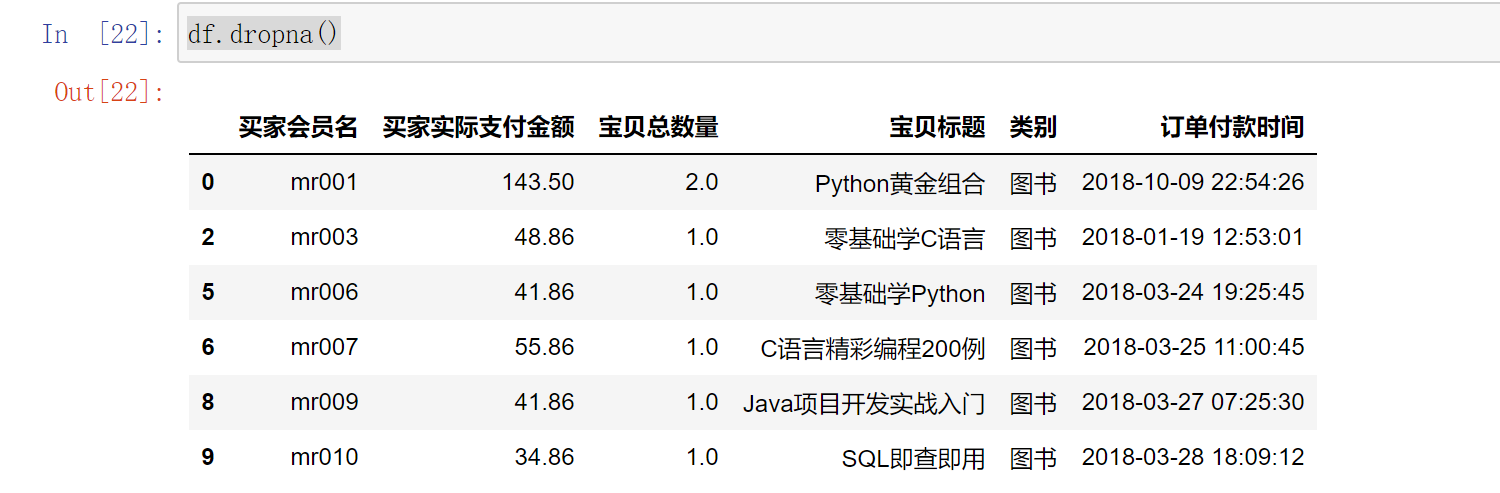3. 缺失值填充处理## 2. 重复值处理

1. 判断每一行数据是否重复(完全相同)

``````df.duplicated()
``````
2. 去除重复的全部数据

``````df.drop_duplicates()
``````
3. 去除指定列的重复数据

``````df.drop_duplicates(["买家会员名"])
``````
4. 保留重复行中的最后一行

``````df.drop_duplicates(["买家会员名"], keep="last")
``````

以上代码中参数 keep 的值有三个。当 keep=“first” 表示保留第一次出现的重复行时，是默认值；当 keep 为另外两个取值 last 和 False 时，分别表示保留最后一次出现的重复行和去除所有的重复行。

5. 直接删除，保留一个副本

``````df.drop_duplicates(["买家会员名","买家支付宝账号"], inplace=False)
``````

inplace=True 表示直接在原来的 DataFrame 对象上删除重复项，而默认值 False 表示删除重复项后再生成一个副本。

## 3. 异常值的检测与处理

1. 根据给定的数据范围进行判断，不在范围内的数据视为异常值。
2. 均方差。在统计学中，如果一个数据分布近似正态分布(数据分布的一种形式，呈钟型，两头低，中间高，左右对称，因其曲线呈钟形)，那么大约 68% 的数据值都会在均值的一个标准差范围内，大约 95% 的数据值会在两个标准差范围内，大约 99.7% 的数据值会在三个标准差范围内。
3. 箱形图。箱形图是显示一组数据分散情况资料的统计图。它可以将数据通过四分位数的形式进行图形化描述，箱形图通过上限和下限作为数据分布的边界。任何高于上限或低于下限的数据都可以认为是异常值，如图所示。• 最常用的方式是删除。
• 将异常值当缺失值处理，以某个值填充。
• 将异常值当特殊情况进行分析，研究异常值出现的原因。

# 八、索引值的设置

## 1. 索引的作用

1. 更方便地查询数据。
2. 使用索引可以提升查询性能。
• 如果索引是唯一的，Pandas 会使用哈希表优化，查找数据的时间复杂度为O(1)。
• 如果索引不是唯一的，但是有序，Pandas 会使用二分查找算法，查找数据的时间复杂度为O(logN)。
• 如果索引是完全随机的，那么每次查询都要扫描数据表，查找数据的时间复杂度为O(N)。
3. 利用索引实现自动的数据对齐功能，如图所示。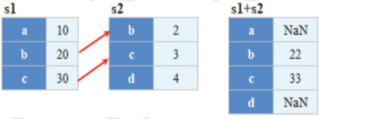实现上述效果，程序代码如下：4. 强大的数据结构
• 基于分类数的索引，提升性能。
• 多维索引，用于 group by 多维聚合结果等。
• 时间类型索引，强大的日期和时间的方法支持。

## 2. 重新设置索引

Pandas 有一个很重要的方法是 reindex，它的作用是创建一个适应新索引的新对象。常用参数说明：

1. labels：标签，可以是数组，默认值为 None。
2. index：行索引，默认值为 None。
3. columns：列索引，默认值为 None。
4. axis：轴，0 表示行；1 表示列，默认值为 None。
6. fill_value：缺失值要填充的数据。如缺失值不用 NaN 填充，用 0 填充，则设置 `fill_value=0` 即可。

1. 对 Series 对象重新设置索引``````print(s1.reindex([1,2,3,4,5], fill_value=0))
``````

``````print(s1.reindex([1,2,3,4,5], method="ffill"))  # 向前填充
print(s1.reindex([1,2,3,4,5], method="bfill"))  # 向后填充
``````

2. 对 DataFrame 对象重新设置索引

``````import pandas as pd  # 导入pandas模块

# 解决数据输出时列名不对齐的问题
pd.set_option("display.unicode.east_asian_width", True)
data = [[110,105,99],[105,88,115],[109,120,130]]
index = ["amo001", "amo003", "amo005"]
columns = ["语文", "数学", "英语"]
df = pd.DataFrame(data=data, index=index, columns=columns)
print(df)
``````

``````print(df.reindex(["amo001","amo002","amo003","amo004","amo005"]))
``````

``````print(df.reindex(columns=["语文","物理","数学","英语"]))
``````

``````print(df.reindex(index=["amo001", "amo002", "amo003", "amo004", "amo005"],columns=["语文","物理","数学","英语"]))
``````

3. 设置某列为索引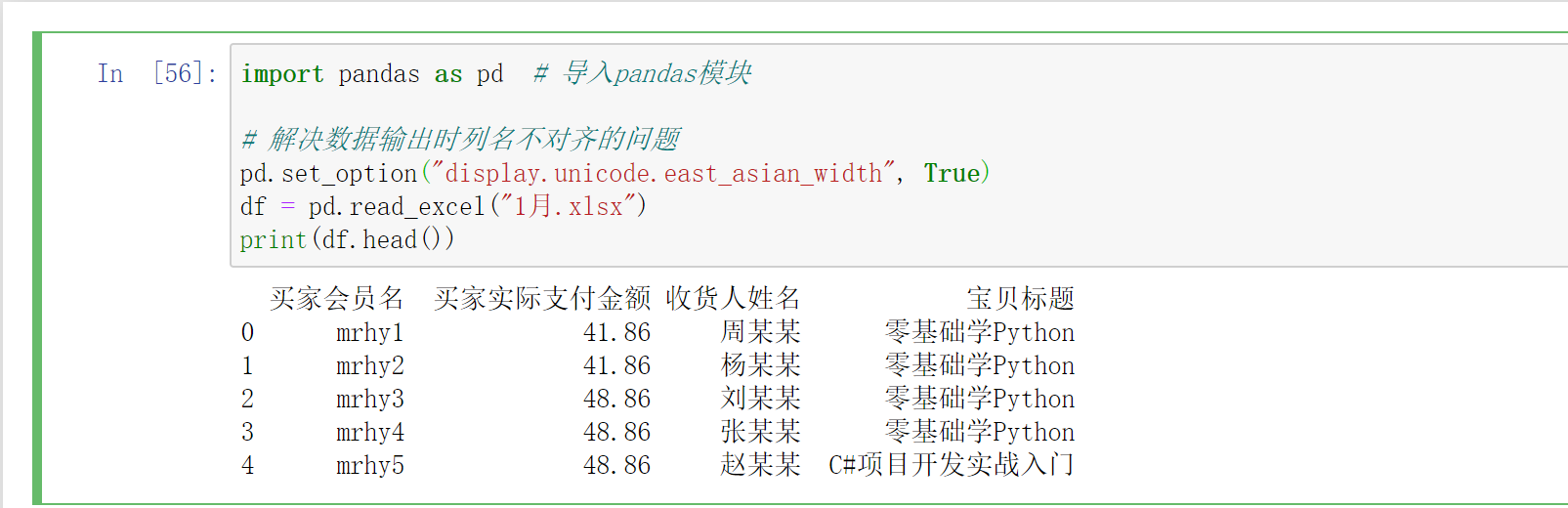4. 数据清洗后重新设置连续的行索引

``````df.dropna().reset_index(drop=True)
``````

# 九、数据排序与排名

## 1. 数据排序

DataFrame 数据排序时主要使用 sort_values() 方法，该方法类似于 SQL 中的 order by 方法。sort_values() 方法可以根据指定行/列进行排序，参数说明：

1. by：要排序的名称列表。
2. axis：轴，0 表示行；1 表示列，默认按行排序。
3. ascending：升序或降序排序，布尔值，指定多个排序可以使用布尔值列表，默认值为 True，升序，如果要降序排列，改为 False 即可。
4. inplace：布尔值，默认值为 False，如果值为 True，则就地排序。
5. kind：指定排序算法，值为 quicksort(快速排序)、mergesort(混合排序) 或 heapsort(堆排)，默认值为 quicksort。
6. na_position：空值(NaN)的位置，值为 first 空值在数据开头，值为 last 空值在数据最后，默认值为 last。
7. ignore_index：布尔值，是否忽略索引，值为 True 标记索引(从 0 开始按顺序的整数值)，值为 False 则忽略索引。

1. 按销量进行降序排列，示例代码如下：

``````import pandas as pd  # 导入pandas模块

excelFile = "mrbook.xlsx"
# 设置数据显示的列数和宽度
pd.set_option("display.max_columns", 500)
pd.set_option("display.width", 1000)

# 解决数据输出时列名不对齐的问题
pd.set_option("display.unicode.ambiguous_as_wide", True)
pd.set_option("display.unicode.east_asian_width", True)
# 按销量列降序排列
df = df.sort_values(by="销量", ascending=False)
print(df)
``````2. 按照图书名称和销量降序排序

``````df = df.sort_values(by=["图书名称", "销量"], ascending=False)
``````

3. 对分组统计数据进行排序

``````df1 = df.groupby(["类别"])["销量"].sum().reset_index()
df2 = df1.sort_values(by="销量", ascending=False)
``````

4. 按行数据进行排序

``````dfrow.sort_values(by=0,ascending=True,axis=1)
``````

## 2. 数据排名

1. axis：轴，0 表示行；1 表示列，默认按行排序。
2. method：表示在具有相同值的情况下所使用的排序方法。设置值如下：
• average：默认值，平均排名。
• min：最小值排名。
• max：最大值排名。
• first：按值在原始数据中的出现的顺序分配排名。
• dense：密集排名，类似最小值排名，但是排名每次只增加 1，即排名相同的数据只占一个名次。
3. numeric_only：对于 DataFrame 对象，如果设置值为 True，则只对数字列进行排序。
4. na_option：空值的排序方式，设置值如下：
1. keep：保留，将空值等级赋值给 NaN 值。
2. top：如果按升序排序，则将最小排名赋值给 NaN 值。
3. bottom：如果按升序排序，则将最大排名赋值给 NaN 值。
5. ascending：升序或降序排序，布尔值，指定多个排序可以使用布尔值列表，默认值为 True。
6. pct：布尔值，是否以百分比形式返回排名，默认值为 False。

1. 对产品销量按顺序进行排名

``````import pandas as pd  # 导入pandas模块

excelFile = "mrbook.xlsx"
# 设置数据显示的列数和宽度
pd.set_option("display.max_columns", 500)
pd.set_option("display.width", 1000)

# 解决数据输出时列名不对齐的问题
pd.set_option("display.unicode.ambiguous_as_wide", True)
pd.set_option("display.unicode.east_asian_width", True)
# 按销量列降序排序
df = df.sort_values(by="销量", ascending=False)
# 顺序排名
df["顺序排名"] = df["销量"].rank(method="first", ascending=False)
print(df[["图书名称","销量", "顺序排名"]])
``````

2. 对产品销量进行平均排名

``````df["平均排名"] = df["销量"].rank(ascending=False)
``````

3. 最小值排名

``````df["销量"].rank(method="min", ascending=False)
``````

4. 最大值排名

``````df["销量"].rank(method="max", ascending=False)
``````好书不厌读百回，熟读课思子自知。而我想要成为全场最靓的仔，就必须坚持通过学习来获取更多知识，用知识改变命运，用博客见证成长，用行动证明我在努力。
如果我的博客对你有帮助、如果你喜欢我的博客内容，请 `点赞``评论``收藏` 一键三连哦！听说点赞的人运气不会太差，每一天都会元气满满呦！如果实在要白嫖的话，那祝你开心每一天，欢迎常来我博客看看。
编码不易，大家的支持就是我坚持下去的动力。点赞后不要忘了 `关注` 我哦！